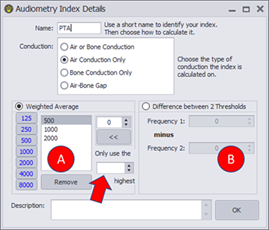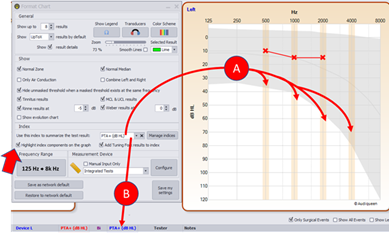Have a look at the instructional video on Formatting.

An index is a numerical value to summarize the test result.  The typical index for pure tone audiometry is the PTA or Pure Tone Index or Fletcher Index.  This is calculated as the average of the air conduction thresholds at 500, 1000 and 2000 Hz.  Other indices may be calculated and  Audiqueen makes it easy for you to create different indices.  For this open the Manage Indices button in the Format window.You first define the name of the index and which conduction you want to use as its basis.  The typical conduction would be air conduction, but for specific  purposes you may be interested in bone conduction or air-bone gap.Then you choose whether to calculate the index as a average of thresholds (A) or a difference between two thresholds (B).  The latter may be interesting, for instance, to calculate a difference between the threshold at 500 Hz and 2000 Hz.  If positive, this may be indicative for hydrops; if negative it may be indicative for presbycusis.  For (A), enter the different thresholds and Audiqueen will calculate the average.  You may also decide to only use the X highest values (arrow).  For instance, if you enter 250, 500, 1000, 2000, 4000 Hz as basis for the index and then select ‘only use the 3 highest’, Audiqueen will take the three worst points out of 5 and it will calculate the average of those 3 points.If you check the checkbox ‘Highlight index components on the graph’ (arrow), Audiqueen indicates which points it has used to calculate the index (A).  Theindex selected in the Index field becomes the one displayed in the grid (B).